### 4.6 Box Plot and Skewed Distributions

Now we have a multitude of numerical descriptive statistics that describe some feature of a data set of values: mean, median, range, variance, quartiles, etc. There are, in fact, so many different descriptors that it is going to be convenient to collect the in a suitable graph. That graph is called the Box Plot.

The Box Plot, sometimes also called "box and whiskers plot", combines the minimum and maximum values (i.e. the range) with the quartiles into on useful graph. It consists of a horizontal line, drawn according to scale, from the minimum to the maximum data value, and a box drawn from the lower to upper quartile with a vertical line marking the median.

To see how it works, it is best to consider an example.

Example: In an earlier example we considered the following cotinine levels of 40 smokers. Draw a box plot for that data.

 0 87 173 253 1 103 173 265 1 112 198 266 3 121 208 277 17 123 210 284 32 130 222 289 35 131 227 290 44 149 234 313 48 164 245 477 86 167 250 491

We already computed the lower and upper quartiles to be Q1 = 86.5 and Q3 = 251.5, respectively. It is easy to see that the minimum is 0 and the maximum data value is 491. A quick computation shows that the median is 170. The corresponding box plot looks therefore as follows: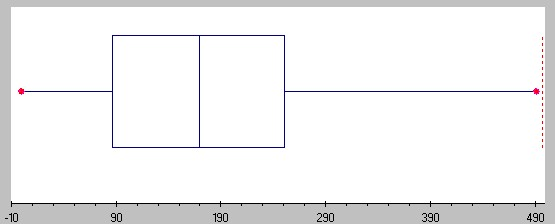You can see that the horizontal line (sometimes called the "whiskers") goes from 0 to 491 (from min to max), while the box extends from 86.5 (= Q1) to 251.5 (= Q3) with a middle vertical line at 170 (the median).

### Drawing a Box Plot with Excel

Unfortunately Excel does not have a nice build-in facility to quickly create a box plot. You could of course use the formulas "max(RANGE)", "min(RANGE)" together with "PERCENTILE(RANGE, 0.25)", "PERCENTILE(RANGE, 0.75)" and "median(RANGE)" and then draw a box plot by hand. However, I found an easy-to-use Excel template that is not quite as convenient as the Data Analysis tools we've been using, but should still be pretty simple and useful.

To use the Excel Box Plot template, click on the icon below to download the file:boxplot.xls

When you open the file, Excel will show you a worksheet with a finished box plot already, and a column on the right in green where you can enter your data. Simply delete the data currently in that column and replace it with your new data. The box plot will update automatically.

Example: Create a box plot for earlier data file on Life Expectancy by country.

We first need to open the Life Expectancy data file - click on the icon below for the data file.life.xls

When the spreadsheet opens up, mark all numeric data in column B (the Life Expectancy column) but not including the column header and copy them to the clipboard (for example, press CTRL-C). Then open the boxplot.xls spreadsheet and position your cursor to the first data value in column M. Paste the copied data values (for example, press CTRL-V) into that column and the box plot will automatically update itself so that you should see the following picture: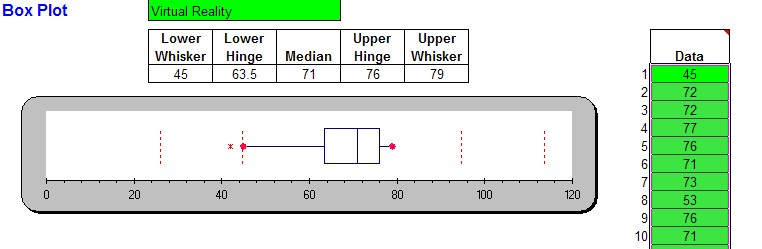### Box Plot and Distributions

In addition to giving you a quick view of the range, the quartiles, and the median, the picture also indicates that if we were to draw a histogram for this data it would look slightly skewed to the left because the box in the box plot is a little towards the left side. In fact, even though the box plot does not directly contain the mean (it only shows the median) it is possible to estimate whether the mean is less than or greater than the median by looking whether the box plot is skewed to the left or to the right.

First, let's look again at histograms and define what we mean by "skewed" histograms (and distributions):

 A histogram (distribution) is called Bell-Shaped or normal if it looks similar to a "bell curve". Most data points fall in the middle, there are few exceptionally small and few exceptionally large values.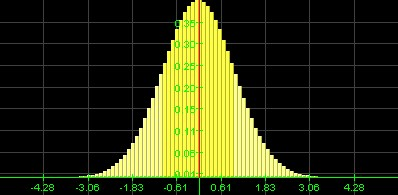Normal Distribution A histogram (distribution) is called skewed to the right if it looks like a bell curve with a longer tail on the right and the mount pushed somewhat to the left. Most data points fall to the left of the middle, there are more exceptionally small than exceptionally large values.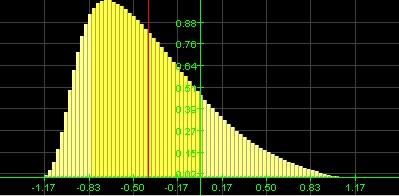Skewed to the right Distribution A histogram (distribution) is called skewed to the left if it looks like a bell curve with a longer tail on the left and the mount pushed somewhat to the right. Most data points fall to the right of the middle, there are more exceptionally large than exceptionally small values.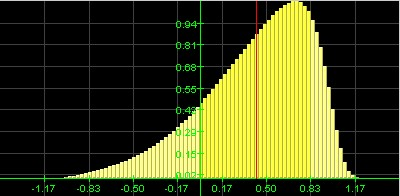Skewed to the left Distribution

You can tell the shape of the histogram (distribution) - in many cases at least - by just looking the box plot, and you can also estimate whether the mean is less than or greater than the median. Recall that the mean is impacted by especially large or small values, even if there are just a few of them, while the median is more stable with respect to exceptional values. Therefore:

• If the distribution is normal, there are few exceptionally large or small values. The mean will be about the same as the median, and the box plot will look symmetric.
• If the distribution is skewed to the right most values are 'small', but there are a few exceptionally large ones. Those exceptional values will impact the mean and pull it to the right, so that the mean will be greater than the median. The box plot will look as if the box was shifted to the left so that the right tail will be longer, and the median will be closer to the left line of the box in the box plot.
• If the distribution is skewed to the left, most values are 'large', but there are a few exceptionally small ones. Those exceptional values will impact the mean and pull it to the left, so that the mean will be less than the median. The box plot will look as if the box was shifted to the right so that the left tail will be longer, and the median will be closer to the right line of the box in the box plot.

As a quick way to remember skewedness:

• longer tail on the left means skewed to the left means mean on the left of median (smaller)
• longer tail on the right means skewed to the right means mean on the right of median (larger)
• tails equally long means normal means mean about equal to median

Example: Here is some (fictitious) data in an Excel sheet for three variables named varA, varB, and varC.distribution-data.xls

Create a box plot for the data from each variable and decide, based on that box plot, whether the distribution of values is normal, skewed to the left, or skewed to the right, and estimate the value of the mean in relation to the median.

One of the data columns has the following box plot and interpretation based on it: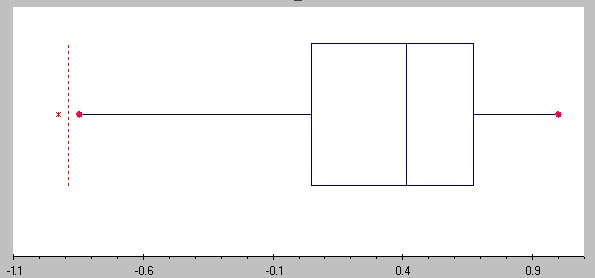Distribution is shifted to the left, the mean should be less than median (the exact numbers are: mean = 0.3319, median = 0.4124).

The other data column has the following box plot and interpretation based on it: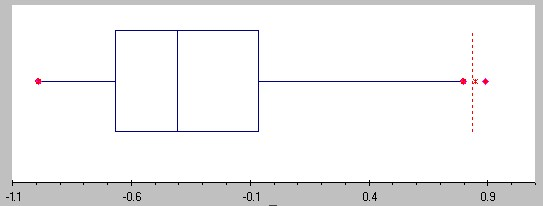Distribution is shifted to the right, the mean should be greater than the median (the exact numbers are: mean = -0.3192, median = -0.4061)

The final data column has the following box plot and interpretation based on it: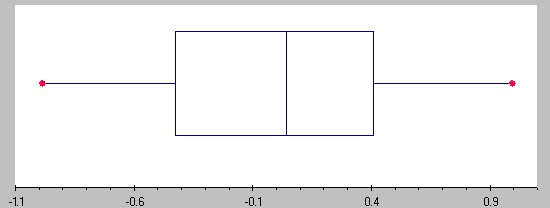Distribution is (approximately) normal, mean and median should be similar (the exact numbers are: mean = 0.013 median = 0.041)

Unfortunately I forgot to write down which of these cases correspond to varA, varB, and varC - can you figure it out? If you need to know the correct answer, just send me an email -:)